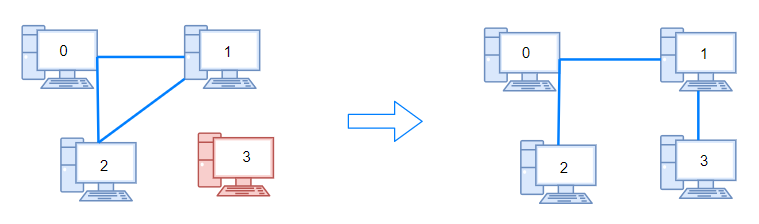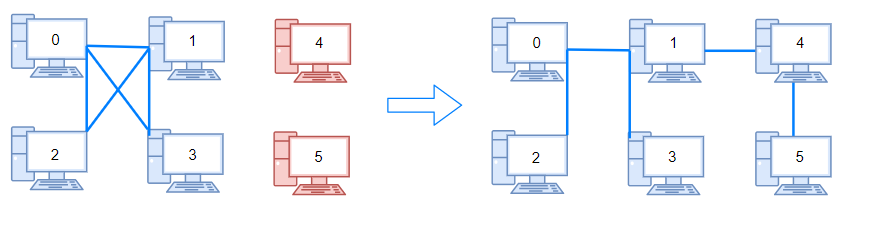# 1319. Number of Operations to Make Network Connected ⭐

Medium
There are `n` computers numbered from `0` to `n - 1` connected by ethernet cables `connections` forming a network where `connections[i] = [ai, bi]` represents a connection between computers `ai` and `bi`. Any computer can reach any other computer directly or indirectly through the network.
You are given an initial computer network `connections`. You can extract certain cables between two directly connected computers, and place them between any pair of disconnected computers to make them directly connected.
Return the minimum number of times you need to do this in order to make all the computers connected. If it is not possible, return `-1`.
Example 1:Input: n = 4, connections = [[0,1],[0,2],[1,2]]
Output: 1
Explanation: Remove cable between computer 1 and 2 and place between computers 1 and 3.
Example 2:Input: n = 6, connections = [[0,1],[0,2],[0,3],[1,2],[1,3]]
Output: 2
Example 3:
Input: n = 6, connections = [[0,1],[0,2],[0,3],[1,2]]
Output: -1
Explanation: There are not enough cables.
Constraints:
• `1 <= n <= 10^5`
• `1 <= connections.length <= min(n * (n - 1) / 2, 105)`
• `connections[i].length == 2`
• `0 <= ai, bi < n`
• `ai != bi`
• There are no repeated connections.
• No two computers are connected by more than one cable.

### 解題

func makeConnected(n int, connections [][]int) int {
if len(connections) < n - 1 {
return -1
}
parents := make([]int, n)
for i := 0; i < n; i++ {
parents[i] = i
}
ans := n - 1 // 連接n台電腦最少需要的線數量
for _, c := range connections {
p0 := findParent(parents, c)
p1 := findParent(parents, c)
if (p0 != p1) {
parents[p0] = p1; // 連通兩台電腦
ans--; // 這條線一開始就是必須的，不能移動
}
}
return ans
}
func findParent(parents []int, i int) int {
if parents[i] == i { // parent 相同 -> 已連通
return i
}
p := findParent(parents, parents[i])
parents[i] = p
return p
}